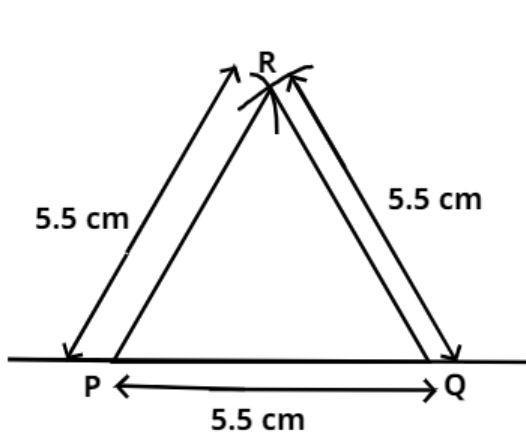Courses
Courses for Kids
Free study material
Offline Centres
MoreLast updated date: 03rd Dec 2023
Total views: 385.2k
Views today: 9.85k

# Construct an equilateral triangle of side 5.5cm.Verified
385.2k+ views
Hint: Use rounder and scale to construct the arc.
Steps of construction: -
$\left( i \right)$ Draw a line segment which is sufficiently long using a ruler.
$\left( {ii} \right)$ Locate points P and Q on it such that $PQ = 5.5cm$.
$\left( {iii} \right)$ With P as center and radius $5.5cm$, draw an arc (see figure).
$\left( {iv} \right)$ With Q as center and radius $5.5cm$, draw another arc cutting the previous arc at R.
$\left( v \right)$ Join PR and QR
PQR is the required equilateral triangle.Note: - In such types of problems the key concept is that always remember the following steps which are stated above, these steps are the basics of the construction, and the required equilateral triangle is shown above.